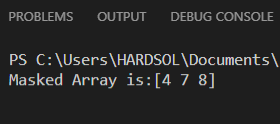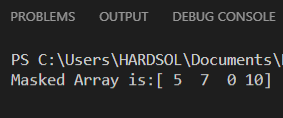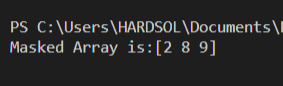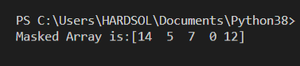GeeksforGeeks App
Open AppBrowser
Continue

# How to mask an array using another array in Python ?

In this article, we will learn how to mask an array using another array in Python. When working with data arrays or data-frames masking can be extremely useful. Masks are an array that contains the list of boolean values for the given condition. The masked array is the arrays that have invalid or missing entries.

Using Masking of arrays we can easily handle the missing, invalid, or unwanted entries in our array or dataset/dataframe. Masking is essential works with the list of Boolean values i.e, True or False which when applied to an original array to return the element of interest, here True refers to the value that satisfies the given condition whereas False refers to values that fail to satisfy the condition.

We can mask the array using another by using the following functions:-

where,

### Steps Required

• Import the library.
• Create a function for masking.
• Masking can be done by following two approaches:-
• Using masked_where() function: Pass the two array in the function as a parameter then use numpy.ma.masked_where() function in which pass the condition for masking and array to be masked. In this we are giving the condition for masking by using one array and masking the another array for that condition.
• Then return the masked from the function.
• Now create the main function
• Create two arrays one for masking another.
• Then call the function as we have created above and pass both the arrays in the function as a parameter and store the result in a variable let named ‘masked’.
• Now for getting the array as a 1-d array we are using numpy.ma.compressed() which passes the masked as a parameter.
• Then print the Masked array.

Example 1: Masking the first array using the second array

In the above example, we are masking the first array using the second array on the basis of the condition that each element of the first array mod 7 is true, those elements which satisfy the condition at that index elements are masked in the first array.

Since we have the array1 = [1,2,4,5,7,8,9] and array2 = [10,12,14,5,7,0,13], we have given the condition array2%7 so in array2 element 14, 7 and 0 satisfies the condition, and they are present at index 2,4 and 5 so at the same index in array1 elements are masked so the resultant array we have [4 7 8].

## Python

 `# importing the library``import` `numpy as np`` ` `# function to create masked array``def` `masking(ar1, ar2):``   ` `  ``# masking the array1 by using array2 ``  ``# where array2 mod 7 is true``  ``mask ``=` `np.ma.masked_where(ar2``%``7``,ar1)``   ` `  ``return` `mask`` ` `# main function``if` `__name__ ``=``=` `'__main__'``:``   ` `  ``# creating two arrays``  ``x ``=` `np.array([``1``,``2``,``4``,``5``,``7``,``8``,``9``])``  ``y ``=` `np.array([``10``,``12``,``14``,``5``,``7``,``0``,``13``])``   ` `  ``# calling masking function to get ``  ``# masked array``  ``masked ``=` `masking(x,y)``   ` `  ``# getting the values as 1-d array which ``  ``# are non masked ``  ``masked_array ``=` `np.ma.compressed(mask)`` ` `  ``# printing the resultant array after masking``  ``print``(f``'Masked Array is:{masked_array}'``)`

Output:Example 2: Masking the second array using the first array

In the above example, we are masking the second array using the first array, giving the condition array1<5 means the elements of array1 which are less than 5 are satisfying the condition and the index of that element will be masked in the second array.

Since we have array1 = [1,2,4,5,7,8,9] and array2 = [10,12,14,5,7,0,13], so in array1 elements 1,2 and 4 are less than 5 these are present at index 0,1 and 2, so this element satisfies the condition so in array2 the elements present at the same index are masked, and we are using the function numpy.ma.compressed() so this function returns the non mask values.  So that we are having [5 7 0 10] after masking.

## Python

 `# importing the library``import` `numpy as np`` ` `# function to create masked array``def` `masking(ar1, ar2):`` ` `    ``# masking the array2 by using array1``    ``# where condition array1 is less than``    ``# 5 is true``    ``mask ``=` `np.ma.masked_where(ar1 < ``5``, ar2)`` ` `    ``return` `mask`` ` ` ` `# main function``if` `__name__ ``=``=` `'__main__'``:`` ` `    ``# creating two arrays``    ``x ``=` `np.array([``1``, ``2``, ``4``, ``5``, ``7``, ``8``, ``9``])``    ``y ``=` `np.array([``10``, ``12``, ``14``, ``5``, ``7``, ``0``, ``13``])`` ` `    ``# calling masking function to get``    ``# masked array``    ``masked ``=` `masking(x, y)`` ` `    ``# getting the values as 1-d array which ``    ``# are non masked``    ``masked_array ``=` `np.ma.compressed(mask)`` ` `    ``# printing the resultant array after masking``    ``print``(f``'Masked Array is:{masked_array}'``)`

Output:Example 3: Masking the first array using the second array though getmask() function

In the above example, for making the mask of the first array using the second array, firstly we are creating the mask of the second array by giving the condition ar2%3 for ar2. Then we are using numpy.ma.getmask() function in which we are passing the result of the created mask, then we are creating the mask of the first array by using numpy.ma.masked_array() in which pass ar1 and pass mask=res_mask which is the mask of array2.

In this way, we can do the masking of one array using another array.

## Python

 `# importing the library``import` `numpy as np`` ` `# function to create masked array``def` `masking(ar1, ar2):`` ` `    ``# creating the mask of array2 where``    ``# condition array2 mod 3 is true``    ``mask ``=` `np.ma.masked_where(ar2 ``%` `3``, ar2)`` ` `    ``# getting the mask of the array``    ``res_mask ``=` `np.ma.getmask(mask)`` ` `    ``# masking the array1 with the result ``    ``# of mask of array2``    ``masked ``=` `np.ma.masked_array(ar1, mask``=``res_mask)`` ` `    ``return` `masked`` ` ` ` `# main function``if` `__name__ ``=``=` `'__main__'``:``     ` `    ``# creating two arrays``    ``x ``=` `np.array([``1``, ``2``, ``4``, ``5``, ``7``, ``8``, ``9``])``    ``y ``=` `np.array([``10``, ``12``, ``14``, ``5``, ``7``, ``0``, ``12``])`` ` `    ``# calling masking function to get masked``    ``# array``    ``masked ``=` `masking(x, y)``    ``masked_array ``=` `np.ma.compressed(masked)`` ` `    ``# printing the resultant array after masking``    ``print``(f``'Masked Array is:{masked_array}'``)`

Output:Example 4: Masking the second array using the first array though getmask() function

In the above example, for making the mask of the second array using the first array, firstly we are creating the mask of the first array by giving the condition ar1<4 for ar1. Then we are using numpy.ma.getmask() function in which we are passing the result of the created mask, then we are creating the mask of the second array by using numpy.ma.masked_array() in which pass ar2 and pass mask=res_mask which is the mask of array1.

In this way, we can do the masking of one array using another array.

## Python

 `# importing the library``import` `numpy as np`` ` `# function to create masked array``def` `masking(ar1, ar2):`` ` `    ``# creating the mask of array2 where``    ``# condition array2 mod 3 is true``    ``mask ``=` `np.ma.masked_where(ar2 ``%` `3``, ar2)`` ` `    ``# getting the mask of the array``    ``res_mask ``=` `np.ma.getmask(mask)`` ` `    ``# masking the array1 with the result of ``    ``# mask of array2``    ``masked ``=` `np.ma.masked_array(ar1, mask``=``res_mask)`` ` `    ``return` `masked`` ` ` ` `# main function``if` `__name__ ``=``=` `'__main__'``:``     ` `    ``# creating two arrays``    ``x ``=` `np.array([``1``, ``2``, ``4``, ``5``, ``7``, ``8``, ``9``])``    ``y ``=` `np.array([``10``, ``12``, ``14``, ``5``, ``7``, ``0``, ``12``])`` ` `    ``# calling masking function to get``    ``# masked array``    ``masked ``=` `masking(x, y)``    ``masked_array ``=` `np.ma.compressed(masked)`` ` `    ``# printing the resultant array after masking``    ``print``(f``'Masked Array is:{masked_array}'``)`

Output:My Personal Notes arrow_drop_up